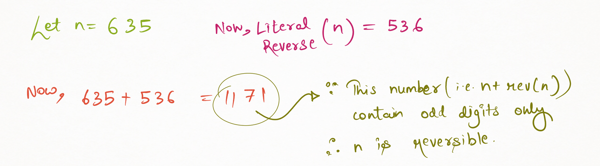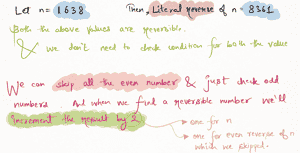Related Tags

project euler
reverse
communitycreator

# ProjectEuler 145: How many reversible numbers are below 1 million

Armaan Nougai

### Problem statement

An integer is called reversible if n + reverse(n) consists only of odd digits. There are 120 such numbers less than 1000.

How many reversible numbers are there that are less than 10^9 (one-billion)?

### Question analysis

Here, the problem has assigned a new definition to the term reversible. We must understand how it works.#### Approach 1

One neophyte way to approach this problem is by simply checking each number to see if it satisfies the condition (i.e., n+rev(n) should contain only odd digits), until one-billion.

##### Optimizations
1. There are no reversible numbers between 10^8 and 10^9.

2. if n is reversible, then n's literal reverse is also reversible. We will avoid checking both of them by just checking one and then adding 2 instead of 1 in the result.#### Approach 2

This solution approach is brain-racking, but this is only because it is long. The upside is that its implementation is a whole lot of small and fast.

Here, we will find out conditions for reversible numbers for the different numbers of digits.

Exactly one of the first and the last numbers must be odd.

##### Condition 1: digit numbers ()
no possible solution


##### Condition 2: digit numbers (a,b)
(a + b) % 2 = 1

a + b < 10, // so that the last digit doesn't pass on its carryover to the first digit, making it even.

Total numbers = 20


##### Condition 3: digit numbers (a,b,c)
(a + c) % 2 = 1

a + c > 10. // since b will be added to itself therefore it will need a carryover from the last digit.

Total numbers = 5*20 = 100


##### Condition 4: digit numbers (a,b,c,d)
(a + d) % 2 = 1

(a + d) < 10

Total numbers = 20*30 = 600
##### Condition 5: digit numbers (a,b,c,d,e)
(d + b) > 10 //since c will be added to itself therefore it will need a carryover from the 4th digit but this will lead the 2nd digit pass to carry over to the 1st digit making it even.

Thus, no possible solution


##### Condition 6: digit numbers (a,b,c,d,e,f)
(f + a) % 2 = 1

(b + e) < 10 // otherwise carryover from 2nd digit will make 1st digit even.

(c + d) < 10 // otherwise carryover from 4th digit will make 3rd digit even.

Total numbers = 20 x 30 x 30 = 18000


##### Condition 7: digit numbers (a,b,c,d,e,f,g)
(a + g) % 2 = 1

// since d will be added to itself, thus need a carryover from the 5th digit.

// Hence, (e + c) > 10

// since (e + c) > 10, 3rd digit will pass carryover to 2nd digit , that means (b + f) % 2 = 0

// Now, the 6th digit is even, to make it odd, 7th digit must be (a + g) > 10 also

Total numbers = 50,000


##### Condition 8: digit numbers (a,b,c,d,e,f,g,h)
(a + h) % 2 = 1

(g + b) < 10 // otherwise it will make the first digit even.

(f + c) < 10 // otherwise it will make the second digit even.

(e + d) < 10 // otherwise it will make the third digit even.

Total numbers = 20 x 30 x 30 x 30 = 540000


##### Condition 9: digit numbers (a,b,c,d,e,f,g,h,i)
// e will be added to itself, but if we keep on going setting up favorable conditions, eventually the first digit will get even.

// So, no possible solutions

### Summary

For this problem, we can get answers just by adding possible solutions for digits (1-9).

But we can also see some pattern here. For example:

// if n is even, then possible reversible numbers of n digits = 20 * 30 ^ (n/2 - 1)


// now if n % 4 = 1, then possible reversible numbers of n digits = 20 x 20 * (20 * 25) ^ (n/4 - 1)


// and for n % 4 = 3, then possible reversible numbers of n digits = 0

### Code

"""
Coded by - Armaan Nougai
"""

def rev(b):
t_rev = 0
while b > 9:
t_rev = t_rev * 10 + b % 10
b //= 10

return t_rev * 10 + b

def oddcheck(v):
while v > 0:
value = v % 10
if value % 2 == 0:
return False
v //= 10
return True

cnt_rev = 0
for j in range(11, 100000000, 2):
if oddcheck(j + rev(j)):
cnt_rev += 2

print(cnt_rev)


RELATED TAGS

project euler
reverse
communitycreator

CONTRIBUTOR

Armaan Nougai
RELATED COURSES

View all Courses

Keep Exploring

Learn in-demand tech skills in half the time